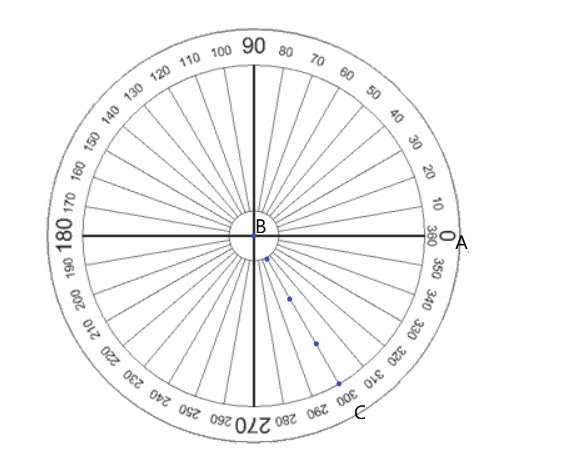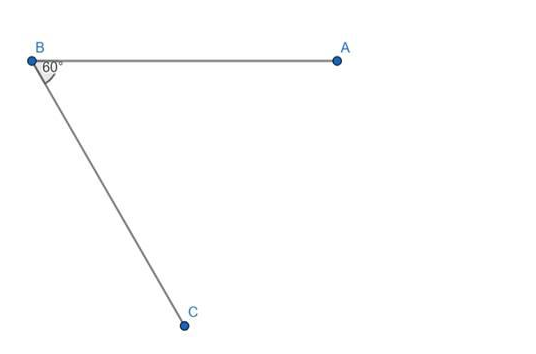# Using a protractor, draw each of the following angles.

Question:

Using a protractor, draw each of the following angles.

$300^{\circ}$

Solution:- Draw a straight line $A B$.

- Place a dot at B. This dot represents the vertex of the angle.

- Place the centre of the protractor at B and the baseline of the protractor along the arm BA.

- Find $300^{\circ}$ on the scale and mark a small dot at the edge of the protractor.

- Join the vertex $B$ to the small dot with a ruler to form the second arm, $B C$, of the angle.

- Mark the angle with a small arc as shown below.